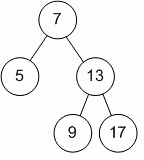favorite We need a little bit of your help to keep things running, click on this banner to learn more
Problems

# Tree Balanced

Given a binary tree. Determine if it is height-balanced. A height-balanced binary tree is defined as a binary tree in which the depth of the two subtrees of every node never differ by more than 1.

Definition of a tree:

// Java
class TreeNode
{
public:
int val;
TreeNode left;
TreeNode right;
TreeNode(int x) {
val = x;
left = NULL;
right = NULL;
};

// C++
class TreeNode
{
public:
int val;
TreeNode *left;
TreeNode *right;
TreeNode(int x) : val(x), left(NULL), right(NULL) {}
};


Implement function isBalanced that returns true if the tree is balanced and false otherwise.

// Java
boolean isBalanced(TreeNode tree)

// C++
bool isBalanced(TreeNode *tree)


#### ExampleFunction isBalanced returns true because tree is balanced.

Time limit 1 second
Memory limit 128 MiB
Author Mykhailo Medvediev